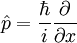# Momentum (physics)

Jump to: navigation, search

Momentum is the "quantity of motion" an object possesses. In classical physics, the linear form of momentum is defined as the product of mass and velocity: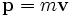Hence, the faster an object goes, or the more mass it possesses, the more momentum it has. Momentum is a vector quantity, and therefore has both a magnitude and direction. It is important to physicists because it is a conserved quantity, making it useful for solving problems.

In common usage, the words "momentum" and "inertia" are sometimes used interchangeably. Inertia is the tendency for a body to resist changes in its motion until and unless a force acts on it.

The motion of an object will continue until something makes it change its motion. A railroad car, once it gets going, will continue its motion for a long time, until the tiny forces of friction cause it to slow down and stop. This can take miles. Even putting on the brakes can take up to mile, because there is so much momentum.

A force in the same direction as the body is moving will increase its speed. A force in the opposite direction will slow it down.

A force coming from the side will cause a deviation from straight-line motion.

An interesting case of a sideways force is a weight on the end of a string (like the Biblical slingshot used by David against Goliath). When you twirl the weight around above your head, the string is pulling the weight toward you - but it never gets any closer! This kind of force is called a centripetal, or center seeking, force.

## Angular momentum

A rotating or orbiting body possesses angular momentum. Like linear momentum, angular momentum is a vector quantity and is conserved. An object's angular momentum changes only when a torque is applied to it. It is defined as: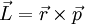Where

•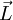is the angular momentum
•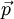is the linear momentum
•is the displacement vector from the origin to the particle

In classical mechanics, the magnitude of the angular momentum of a particle orbiting some origin (such as the earth orbiting the sun) is given by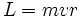where

•is the magnitude angular momentum
•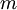is the mass of the particle
•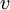is the tangential velocity of the particle
•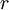is the distance from the particle to the origin

The direction of the angular momentum vector points perpendicularly to the plane formed by the object's orbit, in accordance with the right hand rule.

In addition to orbital angular momentum, the earth has rotational angular momentum due to its spin. The equations for calculating rotational angular momentum depend on the object's moment of inertia, therefore the shape and density of the object and can be given as: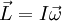Where

•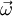is the angular momentum
•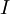is the moment of inertia
•angular velocity of the body

### Generalized momentum

The definition of momentum can be generalized in Lagrangian and Hamiltonian dynamics, towhere L is the Lagrangian andis the velocity. In some cases the generalized momentum is the same as the momentum defined above. For example, for a free particle the Lagrangian equals the kinetic energy and soas above.

## Momentum in Relativity

In relativity, momentum must be redefined for conservation of momentum to be retained. It is defined as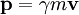whereis the Lorentz factor.

## Momentum in Quantum Mechanics

In quantum mechanics, the operator for momentum,is